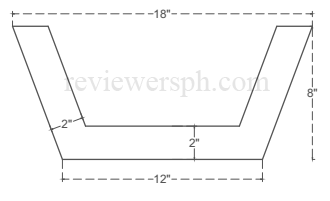### Math Notes

Subjects

#### Solid Geometry Solutions

##### Topics || Problems

Find the area of the rectilinear figure shown, if it is the difference between two isosceles trapezoids whose corresponding sides are parallel.Solution:

Extend lines then we can create 3 similar triangles• Triangle A: Triangle with altitude = 6 and base = z
• Triangle B:Triangle with altitude = 8 and base = 3
• Triangle C: Triangle with altitude = 2 and hypotenuse = x

By AAA theorem we can prove that these triangles are similar. Thus if these triangles are similar we can use ratio and proportion

See a simplified proof/calculation here

$$\frac{6}{z} = \frac{8}{3}$$

$$z = \frac{9}{4}$$

Calculate the hypotenuse of the triangle B: $$8^2 + 3^2 = c^2$$

$$c = \sqrt{73}$$

Ratio and Proportion between triangles B and C.

$$\frac{2}{x} = \frac{8}{\sqrt{73}}$$

$$x = \frac{\sqrt{73}}{4}$$

Calculate the length of $$y$$: $$y = ~18 ~- ~2x ~- ~2z$$

$$y = 18 - (2) \frac{9}{4} - (2) \frac{\sqrt{73}}{4}$$

$$y = \frac{27-\sqrt{73}}{2}$$

Find the value of $$q$$: $$q = 18 - 2x$$

$$q = 18 - (2) \frac{\sqrt{73}}{4}$$

$$q = \frac{36-\sqrt{73}}{2}$$

$$A_{Trap(outside)} = \frac{1}{2}(8)(12+18) = \: 120 \: in^2$$

$$A_{Trap(outside)} = 120 \:in ^2$$

$$A_{Trap(inside)} = \frac{1}{2}(6)(\frac{27-\sqrt{73}}{2}+\frac{36-\sqrt{73}}{2})$$

$$A_{Trap(inside)} = 68.86798876 \:in ^2$$

$$A =120 - 68.86798876 \:in ^2$$

$$A =51.13 \: in ^2$$. Answer